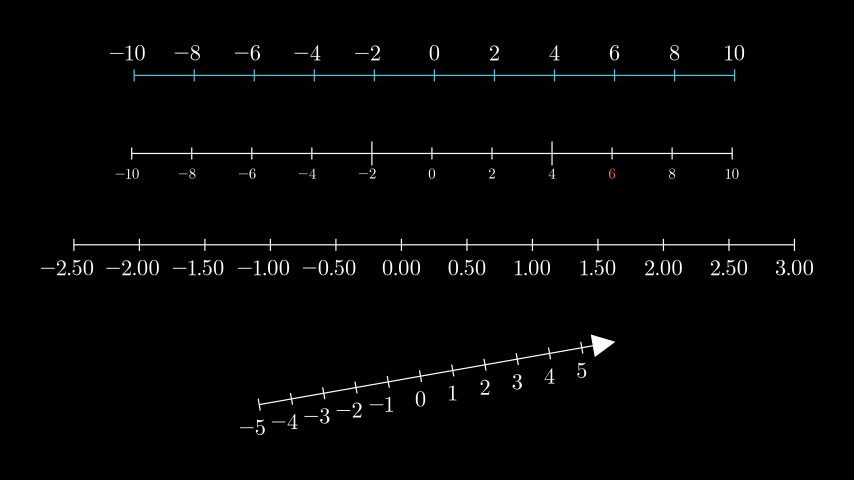# NumberLine¶

Qualified name: `manim.mobject.number\_line.NumberLine`

class NumberLine(x_range=None, length=None, unit_size=1, include_ticks=True, tick_size=0.1, numbers_with_elongated_ticks=None, longer_tick_multiple=2, exclude_origin_tick=False, color='#BBBBBB', rotation=0, stroke_width=2.0, include_tip=False, tip_width=0.25, tip_height=0.25, include_numbers=False, font_size=36, label_direction=array([0., - 1., 0.]), line_to_number_buff=0.25, decimal_number_config=None, numbers_to_exclude=None, numbers_to_include=None, **kwargs)[source]

Creates a number line with tick marks. Number ranges that include both negative and positive values will be generated from the 0 point, and may not include a tick at the min / max values as the tick locations are dependent on the step size.

Parameters
• x_range (Optional[Sequence[float]]) – The `[x_min, x_max, x_step]` values to create the line.

• length (Optional[float]) – The length of the number line.

• unit_size (float) – The distance between each tick of the line. Overwritten by `length`, if specified.

• include_ticks (bool) – Whether to include ticks on the number line.

• tick_size (float) – The length of each tick mark.

• numbers_with_elongated_ticks (Optional[Iterable[float]]) – An iterable of specific values with elongated ticks.

• longer_tick_multiple (int) – Influences how many times larger elongated ticks are than regular ticks (2 = 2x).

• color (colour.Color) – The color of the line.

• rotation (float) – The angle (in radians) at which the line is rotated.

• stroke_width (float) – The thickness of the line.

• include_tip (bool) – Whether to add a tip to the end of the line.

• tip_width (float) – The width of the tip.

• tip_height (float) – The height of the tip.

• include_numbers (bool) – Whether to add numbers to the tick marks. The number of decimal places is determined by the step size, this default can be overridden by `decimal_number_config`.

• size (font) – The size of the label mobjects. Defaults to 36.

• label_direction (Sequence[float]) – The specific position to which label mobjects are added on the line.

• line_to_number_buff (float) – The distance between the line and the label mobject.

• decimal_number_config (Optional[Dict]) – Arguments that can be passed to `DecimalNumber` to influence number mobjects.

• numbers_to_exclude (Optional[Iterable[float]]) – An explicit iterable of numbers to not be added to the number line.

• numbers_to_include (Optional[Iterable[float]]) – An explicit iterable of numbers to add to the number line

• kwargs – Additional arguments to be passed to `Line`.

• exclude_origin_tick (bool) –

• font_size (float) –

Examples

Example: NumberLineExample```from manim import *

class NumberLineExample(Scene):
def construct(self):
l0 = NumberLine(
x_range=[-10, 10, 2],
length=10,
color=BLUE,
include_numbers=True,
label_direction=UP,
)

l1 = NumberLine(
x_range=[-10, 10, 2],
unit_size=0.5,
numbers_with_elongated_ticks=[-2, 4],
include_numbers=True,
font_size=24,
)
[num6] = [num for num in l1.numbers if num.number == 6]
num6.set_color(RED)

l2 = NumberLine(
x_range=[-2.5, 2.5 + 0.5, 0.5],
length=12,
decimal_number_config={"num_decimal_places": 2},
include_numbers=True,
)

l3 = NumberLine(
x_range=[-5, 5 + 1, 1],
length=6,
include_tip=True,
include_numbers=True,
rotation=10 * DEGREES,
)

line_group = VGroup(l0, l1, l2, l3).arrange(DOWN, buff=1)
```
Returns

The constructed number line.

Return type

NumberLine

Parameters
• x_range (Optional[Sequence[float]]) –

• length (Optional[float]) –

• unit_size (float) –

• include_ticks (bool) –

• tick_size (float) –

• numbers_with_elongated_ticks (Optional[Iterable[float]]) –

• longer_tick_multiple (int) –

• exclude_origin_tick (bool) –

• color (colour.Color) –

• rotation (float) –

• stroke_width (float) –

• include_tip (bool) –

• tip_width (float) –

• tip_height (float) –

• include_numbers (bool) –

• font_size (float) –

• label_direction (Sequence[float]) –

• line_to_number_buff (float) –

• decimal_number_config (Optional[Dict]) –

• numbers_to_exclude (Optional[Iterable[float]]) –

• numbers_to_include (Optional[Iterable[float]]) –

Methods

 `add_labels` Adds specifically positioned labels to the `NumberLine` using a `dict`. `add_numbers` `add_ticks` `create_label_tex` Checks if the label is a `float`, `int` or a `str` and creates a `MathTex`/`Tex` label accordingly. `decimal_places_from_step` `get_labels` `get_number_mobject` `get_number_mobjects` `get_tick` `get_tick_marks` `get_tick_range` `get_unit_size` `get_unit_vector` `n2p` Abbreviation for number_to_point `number_to_point` `p2n` Abbreviation for point_to_number `point_to_number` `rotate_about_number` `rotate_about_zero`

Attributes

 `animate` Used to animate the application of a method. `animation_overrides` `depth` The depth of the mobject. `height` The height of the mobject. `width` The width of the mobject.

Adds specifically positioned labels to the `NumberLine` using a `dict`.

Parameters

dict_values (Dict[float, Union[str, float, Mobject]]) –

static create_label_tex(label_tex)[source]

Checks if the label is a `float`, `int` or a `str` and creates a `MathTex`/`Tex` label accordingly.

Parameters

label_tex (The label to be compared against the above types.) –

Returns

The label.

Return type

`Mobject`

n2p(number)[source]

Abbreviation for number_to_point

Parameters

number (float) –

Return type

numpy.ndarray

p2n(point)[source]

Abbreviation for point_to_number

Parameters

point (Sequence[float]) –

Return type

float